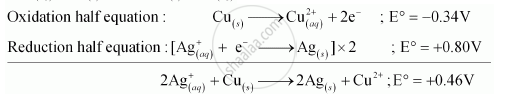CBSE (Science) Class 11CBSE
Share

# Using the Standard Electrode Potentials Given in the Table 8.1, Predict If the Reaction Between the Following is Feasible: Ag+(Aq) and Cu(S) - CBSE (Science) Class 11 - Chemistry

ConceptRedox Reactions in Terms of Electron Transfer Reactions - Introduction

#### Question

Using the standard electrode potentials given in the Table 8.1, predict if the reaction between the following is feasible:

Ag+(aq) and Cu(s)

#### Solution

The possible reaction between Ag_((aq))^+ + Cu_((s)) is given by,

2Ag_((aq))^+ + Cu_(s) -> 2Ag_((s)) + Cu_((aq))^(2+)E° positive for the overall reaction is positive. Hence, the reaction between Ag_((aq))^+ and Cu_((s)) is feasible.

Is there an error in this question or solution?

#### APPEARS IN

NCERT Solution for Chemistry Textbook for Class 11 (2018 to Current)
Chapter 8: Redox Reactions
Q: 26.2 | Page no. 274
Solution Using the Standard Electrode Potentials Given in the Table 8.1, Predict If the Reaction Between the Following is Feasible: Ag+(Aq) and Cu(S) Concept: Redox Reactions in Terms of Electron Transfer Reactions - Introduction.
S